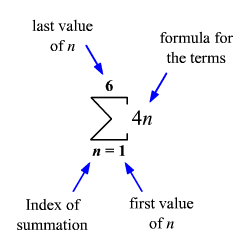# Sigma Notation of a Series

A series can be represented in a compact form, called summation or sigma notation. The Greek capital letter, $\sum$ , is used to represent the sum.

The series $4+8+12+16+20+24$ can be expressed as $\underset{n=1}{\overset{6}{\sum }}4n$ . The expression is read as the sum of $4n$ as $n$ goes from $1$ to $6$ . The variable $n$ is called the index of summation.To generate the terms of a series given in sigma notation, successively replace the index of summation with consecutive integers from the first value to the last value of the index.

To generate the terms of the series given in sigma notation above, replace $n$ by $1,2,3,4,5,\text{\hspace{0.17em}}\text{\hspace{0.17em}}\text{and}\text{\hspace{0.17em}}\text{\hspace{0.17em}}6$ .

$\begin{array}{l}\underset{n=1}{\overset{6}{\sum }}4n=4\left(1\right)+4\left(2\right)+4\left(3\right)+4\left(4\right)+4\left(5\right)+4\left(6\right)\\ \text{\hspace{0.17em}}\text{\hspace{0.17em}}\text{\hspace{0.17em}}\text{\hspace{0.17em}}\text{\hspace{0.17em}}\text{\hspace{0.17em}}\text{\hspace{0.17em}}\text{\hspace{0.17em}}\text{\hspace{0.17em}}\text{\hspace{0.17em}}\text{\hspace{0.17em}}\text{\hspace{0.17em}}\text{\hspace{0.17em}}=4+8+12+16+20+24\\ \text{\hspace{0.17em}}\text{\hspace{0.17em}}\text{\hspace{0.17em}}\text{\hspace{0.17em}}\text{\hspace{0.17em}}\text{\hspace{0.17em}}\text{\hspace{0.17em}}\text{\hspace{0.17em}}\text{\hspace{0.17em}}\text{\hspace{0.17em}}\text{\hspace{0.17em}}\text{\hspace{0.17em}}\text{\hspace{0.17em}}=84\end{array}$

The sum of the series is $84$ .

To learn more about ${S}_{n}$ notation, view this lesson: Sum of the First n Terms of a Series